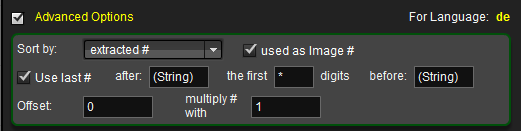In addition and as summary of the settings already discussed, special requirements can usually be resolved with a combination of the following options.

### Extract Number from File Name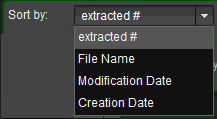If `extracted #` is selected, some special options are available to extract the correct digits and manulipate them.

#### used as image #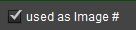Numbers can be interpreted as image or page number.
In case of `used as Image #` selected, The associated page number is calculated by mz3Tool depending on the book type.
For single pages: The page number is the same as the image number.
For double pages: The page number is calculated according to the following formula:

Page number=(picture number – 1) * 2

#### Use Last Number

If this checkbox is set, in case of several blocks of digits are separated by non digit characters, the last block of digits is interpreted as page/image number.
This default setting is useful if the page/image number and other digits like date, issue number or alike are used also. And if those blocks of digits are separated by the same delimiter like a “-”.
Example:

book-4-20190324-43.jpg

With “4” as book index, “20190324” as date and “43” as page number.
If `use last #` is active, “43” will be used as page/image number.

#### Start Search After

If the page/image number e.g. must be extracted from the middle of the name, or from different different positions in the file name, @Use Last #” does not help anymore.
In that case a character pattern can be defined that preceeds the digits of interest.
Often patterns like:

xxx_pg_0001_xxx or xxx_page_1_xxx

are used. Then “pg_” or “page_” can be defined as start pattern, and regardless of the position, the page/image number is found and interpreted correctly.

#### the first nn digits

if not all digits of the filtered block of digits should be used, the length, i.e. number of digits used, can be defined.
This is helpful if e.g. a “0” follows the desired number, i.e. in cases where the page/image number is incremented in steps of 10.

Example: catalog2018Q4_page_00030_en.jpg
The number “0003” can be found with the following settings:

• `after`: Page
• `the first`: 4 `digits`

#### before

The definition of a block of characters that must follow the number to be filtered can als be used.
In case the filtered string still contains more than one block of digits, this option helps specifying the block of interest.
also if fixed trailing numbers hould be excluded fomr the block of digits this can be useful.

Example:

any_123-456_789#12000_en.jpg

If the number to be extracted is 120, the setting `before` 00_en will achieve that.

### Use Sort Index as Image/Page Number

If as sort field

• File Name
• Modification Date
• Creation Date

is selected, the sort index is used as page/image number.
That index can be modified in adding an offset and by multiplication with a number.

### General Settings

Overwrite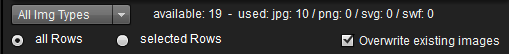If page images already exist on the book pages (page occupied with a previous page image), this checkbox must be activated so that the old page image can be overwritten.

Offset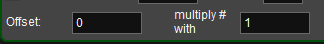The calculated page number, whether determined via a direct assignment of the picture number <-> page number or the index as result of a sort, can be corrected by a fixed “offset” (positive or negative!).

The calculated page number is:

new page number = current number + offset

Multiply # with
The calculated page number is:

new page number = current number * multiplier

The multiplier can be a fractional number like 0.5. This acts like a division by 2.
The multiplier is applied before a potential offset

Image type selection (ALL / JPG / PNG / SWF / SVG)
From a drop-down list the file type to be used can be selected.

If “All” is selected, all files will be used independent on the file type.

### Satus Messages

OK extracted
This image will be used, the page number was successfully extracted from the file name

OK: Index
This image will be used; the page number was built out of the index after a sort of the selected field.

Page blocked
There was already a Page Image added to the page with the calculated page number.
Setting `overwrite` can solve this.

no digits
The file name does not contain any digits, but `extract #` was selected.
invalid
This is shown if a string was filtered that does not contain any digit, or the filtered digitis result in a negative number,

out of range
This status information indicates that the extracted numbers would result in page numbers that are outside of the valid page range of the book

double pgNbr
Shown as status information if the actual settings result in double page numbers

Revision: 29